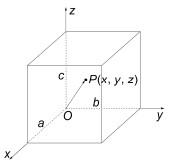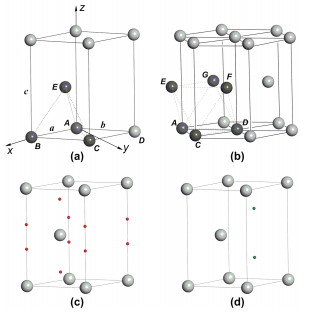## Applications of Centroid Fractional Coordinates in Locating Interstices in Close Packings of Equal Spheres,, , , , ,,

 基金资助: 国家自然科学基金.  21503191中国博士后科学基金.  2015M572115

 Fund supported: 国家自然科学基金.  21503191中国博士后科学基金.  2015M572115Abstract

Deep understanding of the nature and characteristics of close packings of equal spheres is fundamental for further study towards structure and property of metallic crystals. And the knowledge to number and distribution of various interstices in close packings of equal spheres is very important to help illustrate the structure and property of ionic crystals. However, the diversity and complexity of the crystal structures make it difficult in teaching and learning structural chemistry. In this paper, based on discussions on the three most common close packing models (A1, A2, A3), a method to locate centers of interstices according to calculating the centroid fractional coordinates (CFC) of particles constructing these interstices was introduced. In addition, the way using CFCs to calculated distance between vertex and interstice center and the shortest distance from interstice center to surface of the packing sphere was also illustrated in detail. Compared with the traditional solid geometry method, the CFC method is demonstrated to be much simpler, easier to learn, and most importantly, helpful for understanding number and distribution of various interstices in close packings.

Keywords： Interstice ; Fractional coordinate ; Centre of mass ; Close packing

ZHANG Wenjing, TANG Huabiao, ZHU Yanyan, WEI Donghui, LIU Chunmei, TANG Mingsheng. Applications of Centroid Fractional Coordinates in Locating Interstices in Close Packings of Equal Spheres. University Chemistry[J], 2018, 33(9): 95-104 doi:10.3866/PKU.DXHX201802035

## 1 分数坐标和质心坐标

### 图1(x, y, z)即为质心的坐标。

### 图2#### 2.1.1 立体几何法

$\begin{array}{l}AF = \frac{{\sqrt 3 }}{2}AB = \frac{{\sqrt 3 }}{2} \cdot 2R = \sqrt 3 R\\GF = \frac{1}{3}CF = \frac{1}{3}AF = \frac{{\sqrt 3 }}{3}R\\AG = {(A{F^2} - G{F^2})^{\frac{1}{2}}} = [{(\sqrt 3 R)^2} - {(\frac{{\sqrt 3 }}{3}R)^2}){]^{\frac{1}{2}}} = \frac{{2\sqrt 6 }}{3}R\end{array}$

$AO = \frac{3}{4}AG = \frac{{\sqrt 6 }}{2}R$

$OG = \frac{1}{4}AG = \frac{{\sqrt 6 }}{6}R$

$d = AO - R = \frac{{\sqrt 6 }}{2}R - R \approx 0.225R$

$\begin{array}{l}CD = 2R = \frac{{\sqrt 2 }}{2}a\\R = \frac{{\sqrt 2 }}{4}a\end{array}$

$AO = \frac{{\sqrt 6 }}{2}R = \frac{{\sqrt 3 }}{4}a$

$d = \frac{{\sqrt 6 }}{2}R - R = (\frac{{\sqrt 3 }}{4} - \frac{{\sqrt 2 }}{4})a$

$AO = OB = \frac{{\sqrt 2 }}{2}AB = \frac{{\sqrt 2 }}{2} \cdot 2R = \sqrt 2 R$

$d = AO - R = \sqrt 2 R - R \approx 0.414R$

$AO = \sqrt 2 R = \frac{1}{2}a$

$d = \sqrt 2 R - R = (\frac{1}{2} - \frac{{\sqrt 2 }}{4})a$

#### 2.1.2 质心坐标法

##### 2.2 A2型(体心立方密堆积)

A1型密堆积中的多面体空隙均为正多面体空隙，而A2型密堆积中的空隙多面体边长不相等，均为变形多面体(图3(a)3(b))，利用立体几何方法求解将更为困难，而质心坐标方法的推导步骤依然不变，具体如下：

### 图3##### 2.2.1 立体几何法

$AO = \frac{3}{4}AG = \frac{3}{4} \cdot \frac{{\sqrt 5 }}{3}a = \frac{{\sqrt 5 }}{4}a$

A2型堆积是一种非最密堆积，圆球只在C3轴(体对角线)方向上相互接触，因此有如下关系式成立：

$\frac{{\sqrt 3 }}{2}a = 2R$

$d = AO - R = \frac{{\sqrt 5 }}{4} \cdot \frac{4}{{\sqrt 3 }}R - R \approx 0.291R$

$AO = \frac{1}{2}a = \frac{1}{2} \cdot \frac{4}{{\sqrt 3 }}R = \frac{{2\sqrt 3 }}{3}R$

$OE = \frac{{\sqrt 2 }}{2}a = \frac{{\sqrt 2 }}{2} \cdot \frac{4}{{\sqrt 3 }}R = \frac{{2\sqrt 6 }}{3}R$

$d = AO - R = \frac{{2\sqrt 3 }}{3}R - R \approx 0.154R$

##### 2.2.2 质心坐标法

###### 2.3.1 立体几何法

A3型等径圆球密堆积是最密堆积，形成的空隙均为正多面体空隙，立体几何法抽象的模型与A1型相同(图2(c)(d))，因此有关立体几何法推算空隙的具体过程此处不再赘述。但是需要注意的是，A3型六方最密堆积中晶胞参数a与等径圆球半径R的关系(a = 2R)与A1型立方最密堆积($(R = \frac{{\sqrt 2 }}{4}a)$)中不同，因此在用晶胞参数表示中心到顶点的距离等几何量时的结果也有差异。

A3型晶胞中四面体空隙中心到顶点的距离和中心到球面的最短距离分别为

$AO = \frac{{\sqrt 6 }}{2}R = \frac{{\sqrt 6 }}{4}a$

$d = \frac{{\sqrt 6 }}{2}R - R = (\frac{{\sqrt 6 }}{4} - \frac{1}{2})a$

$AO = \frac{{\sqrt 2 }}{2}a$

$d = \sqrt 2 R - R = (\frac{{\sqrt 2 }}{2} - \frac{1}{2})a$

### 图4## 参考文献 原文顺序 文献年度倒序 文中引用次数倒序 被引期刊影响因子

2009, (10), 8.

/

 〈〉